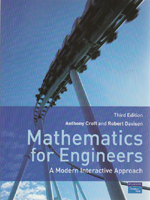Mathematics for Engineers, 3rd edition

A Modern Interactive Approach

By Anthony Croft and Robert Davison
September 2008
Pearson / Prentice Hall
Distributed By Trans-Atlantic Publications
ISBN: 97801320515691173 pages, Illustrated
\$115.00 Paper Original

Understanding key mathematical concepts and applying them successfully to solve problems are vital skills that all engineering students must acquire. Mathematics for Engineers teaches, develops and nurtures these skills. Practical, informal, and accessible, it beings with the foundations and gradually builds upon this knowledge as it introduces more complex concepts until you have learned everything you will need for your first year engineering maths course, together with introductory material for even more advanced topics.

Key Features:

--Hundreds of Interactive examples are provided in the context of various engineering disciplines, so you are able to both engage with the problems and also see the relevance of the maths to your wider studies
--A wealth of practice and revision exercises with solutions help test your knowledge
--Key points and important results are highlighted throughout
--Computer and calculator examples and exercises are incorporated in relevant sections
Specimen examination papers give further opportunity to practise for high stake assessments
--A foundation section gives you a firm base in arithmetic, the building block of many high level mathematical topics
--An accompanying website is available, which contains supporting resources for both students and teachers.

Contents:

Guided Tour

Publisher's acknowledgements

Preface

Using mathematical software packages

1. Arithmetic
2. Fractions
3. Decimal numbers
4. Percentage and ratio
5. Basic algebra
6. Functions
7. Polynomial equations, inequalitites, partial fractions and proportionality
8. Logartihms and exponentials
9. Trigonometry
10. Further trigonometry
11. Complex numbers
12. Matrices and determinants
13. Using matrices and determinants to solve equations
14. Vectors
15. Differentiation
16. Techniques and applications of differentiation
17. Integration
18. Applications of integration
19. Sequences and series
20. Differential equations
21. Functions of more than one variable and partial differentiation
22. The Laplace transform
23. Statistics and probability
24. An introduction to Fourier series and the Fourier transform

Typical examination papers

Appendix: SI units and prefixes